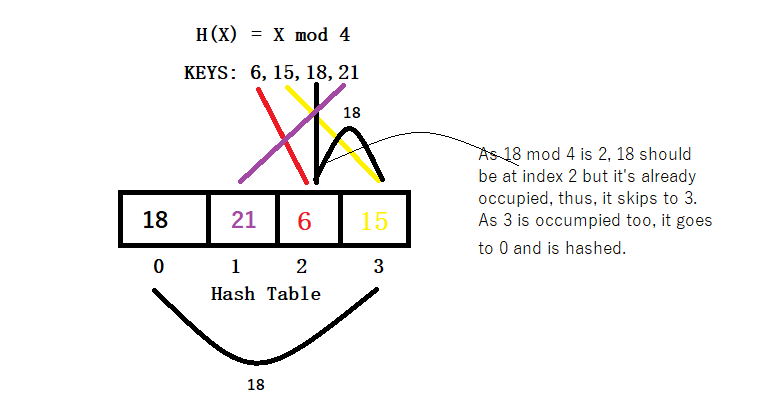Problem title
Difficulty
Avg time to solve

All substrings
Moderate
--
Detect Cycle in a Undirected Graph
Hard
50 mins
Number Of Pairs With Given Sum
Moderate
39 mins
Moderate
30 mins
Maximum Width In Binary Tree
Moderate
38 mins
Distance between two nodes of a Tree
Moderate
25 mins
Yet another Pattern
Easy
15 mins
Sum Of Zeroes
Easy
10 mins
Count Frequency
Easy
15 mins
Left Sum
Easy
10 mins11

# Linear Probing

Difficulty: EASY
Avg. time to solve
15 min
Success Rate
85%

Problem Statement

#### You can refer to the example given below:#### Note:

``````1. Consider ‘0’ based indexing.
``````
##### Input format:
``````The first line of input contains an integer ‘T’ denoting the number of test cases.

The first line of each test case contains a positive integer ‘N’ representing the size of array KEYS.

The second line of each test case contains ‘N’ space-separated non-negative integers representing elements of the array KEYS.
``````
##### Output format :
``````For each test case, return an array 'HASH_TABLE’ of size ‘N’ where HASH_TABLE[ i ] is the element from the given array KEYS which is mapped to the index i.
``````
##### Note:
``````You do not need to print anything, it has already been taken care of. Just implement the given function.
``````
##### Constraints:
``````1 <= T <= 10
1 <= N <= 500
0 <= KEYS[ i ] <= 10^9

Time limit: 1 sec
``````
##### Sample Input 1:
``````2
5
5 3 2 6 4
4
1 5 3 7
``````
##### Sample Output 1:
``````5 6 2 3 4
7 1 5 3
``````
##### Explanation
``````For test case 1:
H(X) = X mod 5
All the numbers have unique hash values so there is no collision. Just map each index to its hash index.

For test case 2:
H(1) = 1L index 1 is unoccupied so we will map index 1 with 1.
H(5) = 1: but, index 1 is occupied, so, we will use index 2 which is unoccupied, and will map index 2 with 5.
H(3) = 3: index 3 is unoccupied so we will map index 3 with 3.
H(7) = 3, but index 3 is occupied so we will use index 0 which is unoccupied and will map index 0 with 7.
``````
##### Sample Input 2:
``````2
6
4 7 8 1 2 5
3
6 7 10
``````
##### Sample Output 2:
``````5 7 8 1 4 2
6 7 10
``````Console